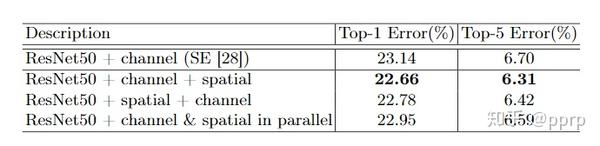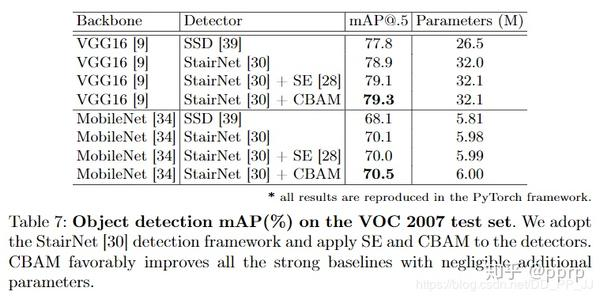# 【CV 中的 Attention 机制】简单而有效的 CBAM 模块

## 1. 什么是注意力机制？

• 空间注意力机制：对空间进行掩码的生成，进行打分，代表是Spatial Attention Module
• 混合域注意力机制：同时对通道注意力和空间注意力进行评价打分，代表的有BAM, CBAM

## 2. CBAM模块的实现

CBAM全称是Convolutional Block Attention Module, 是在ECCV2018上发表的注意力机制代表作之一。本人在打比赛的时候遇见过有人使用过该模块取得了第一名的好成绩，证明了其有效性。

### 2.1 通道注意力机制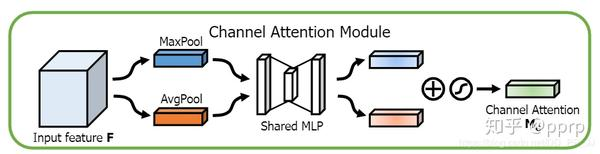class ChannelAttention(nn.Module):
def __init__(self, in_planes, rotio=16):
super(ChannelAttention, self).__init__()

self.sharedMLP = nn.Sequential(
nn.Conv2d(in_planes, in_planes // ratio, 1, bias=False), nn.ReLU(),
nn.Conv2d(in_planes // rotio, in_planes, 1, bias=False))
self.sigmoid = nn.Sigmoid()

def forward(self, x):
avgout = self.sharedMLP(self.avg_pool(x))
maxout = self.sharedMLP(self.max_pool(x))
return self.sigmoid(avgout + maxout)

### 2.2 空间注意力机制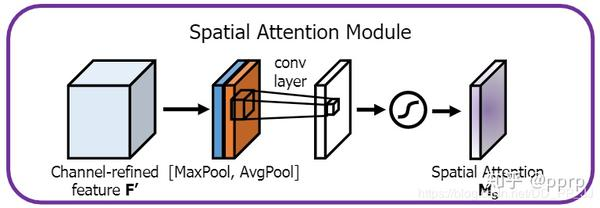class SpatialAttention(nn.Module):
def __init__(self, kernel_size=7):
super(SpatialAttention, self).__init__()
assert kernel_size in (3,7), "kernel size must be 3 or 7"
padding = 3 if kernel_size == 7 else 1

self.sigmoid = nn.Sigmoid()

def forward(self, x):
avgout = torch.mean(x, dim=1, keepdim=True)
maxout, _ = torch.max(x, dim=1, keepdim=True)
x = torch.cat([avgout, maxout], dim=1)
x = self.conv(x)
return self.sigmoid(x)

### 2.3 Convolutional bottleneck attention module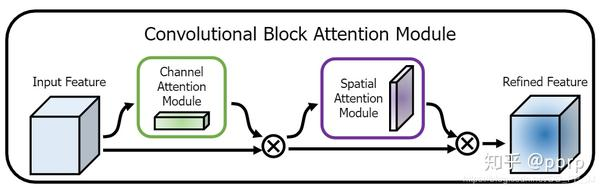class BasicBlock(nn.Module):
expansion = 1
def __init__(self, inplanes, planes, stride=1, downsample=None):
super(BasicBlock, self).__init__()
self.conv1 = conv3x3(inplanes, planes, stride)
self.bn1 = nn.BatchNorm2d(planes)
self.relu = nn.ReLU(inplace=True)
self.conv2 = conv3x3(planes, planes)
self.bn2 = nn.BatchNorm2d(planes)
self.ca = ChannelAttention(planes)
self.sa = SpatialAttention()
self.downsample = downsample
self.stride = stride
def forward(self, x):
residual = x
out = self.conv1(x)
out = self.bn1(out)
out = self.relu(out)
out = self.conv2(out)
out = self.bn2(out)
out = self.ca(out) * out  # 广播机制
out = self.sa(out) * out  # 广播机制
if self.downsample is not None:
residual = self.downsample(x)
out += residual
out = self.relu(out)
return out

class cbam(nn.Module):
def __init__(self, planes)：
self.ca = ChannelAttention(planes)# planes是feature map的通道个数
self.sa = SpatialAttention()
def forward(self, x):
x = self.ca(out) * x  # 广播机制
x = self.sa(out) * x  # 广播机制

## 3. 在什么情况下可以使用？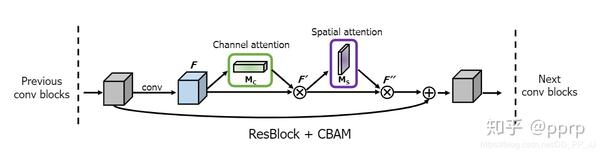1. 如何更有效地计算channel attention\?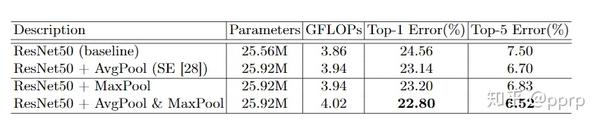1. 如何更有效地计算spatial attention\?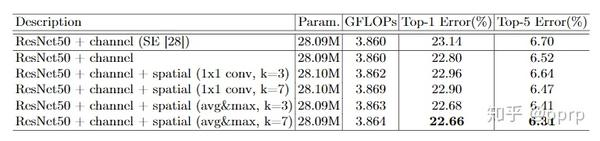1. 如何组织这两个部分？